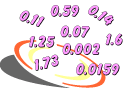# Multiplying and Dividing Decimals

Multiplying and Dividing Decimals is a unit of instruction that shows students how to estimate decimal products and quotients, multiply and divide decimals by whole numbers, and to multiply and divide decimals by decimals. Other topics include rounding decimal quotients and solving word problems. Try our sample lessons below, or browse other units.Topics Description Estimating Products Students are shown how to estimate decimal products using various strategies. Multiplying Decimals and Whole Numbers Students are shown how to multiply decimals by whole numbers. Also examined are overestimates, underestimates, and estimation to determine if an answer is reasonable. Multiplying Decimals Step-by-step examples for multiplying decimals are presented. A structured approach that includes estimating the product, multiplying, and then comparing the product with the estimate is emphasized. Estimating Quotients Students are shown how to estimate decimal quotients using compatible numbers. Dividing Decimals by Whole Numbers Step-by-step examples for dividing decimals by whole numbers are presented. A structured approach that includes estimating the quotient, dividing, and then comparing the quotient with the estimate is emphasized. Rounding Quotients Students will learn how to divide decimals by whole numbers, and then round the quotient to a designated place. Dividing Decimals by Decimals Step-by-step examples for dividing decimals are presented. Students learn to convert the divisor to a whole number by multiplying both the divisor and the dividend by the same power of ten. Solving More Decimal Word Problems Students are shown how to solve decimal word problems using the concepts learned in this unit. Practice Exercises To complete 10 additional exercises as practice. To assess students' understanding of all decimal concepts learned so far. Challenge Exercises To solve 10 additional problems that challenge students' understanding of all decimal topics covered in this unit. To hone students' problem-solving skills. Solutions To review complete solutions to all exercises presented in this unit. Includes the problem, step-by-step solutions, final answer for each exercise. Learning Objectives Learn how to estimate Decimal Products and Quotients. Multiply and Divide Decimals by Whole Numbers and by Decimals. Round Decimal Quotients, Solve Word Problems.